# Brownian local time

(diff) ← Older revision | Latest revision (diff) | Newer revision → (diff)

Let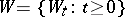be a standard Wiener process (or, in other words, Brownian motion) living on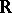and started at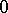. The random set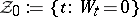, the so-called zero set of the Brownian path, is almost surely perfect (i.e. closed and dense in itself), unbounded and of Lebesgue measure. The complement of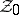is a countable union of open intervals.

A remarkable result of P. Lévy ([a6], [a7]) is that there exists a non-decreasing (random) function determined bywhich is constant on the open intervals in the complement ofand which has every point inas a (left and/or right) strict increase point. This function is called the Brownian local time (at). It is clear that a similar construction can be made at any point.

The existence of the local time can be deduced from the fact (also due to Lévy) that the processes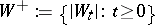and, where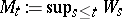, are identical in law. Indeed, for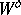the function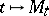has the desired properties of local time; for the proof that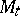, for a given, is determined by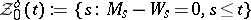, see [a4]. Because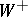andare identical in law, there exists a function with corresponding properties connected to.

Let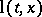be the Brownian local time atat time. Then almost surely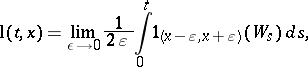and this leads to the occupation-time formula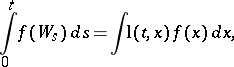whereis a Borel-measurable function (cf. also Borel function).

As seen above,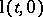can be viewed as the measure of the zero set. In fact, it has been proved in [a11] and [a9] thatis the random Hausdorff-measure (cf. also Hausdorff measure) of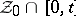with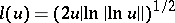.

Introduce for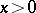the right-continuous inverse ofby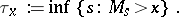By the strong Markov property (cf. Markov property) and spatial homogeneity of Brownian motion, the processis increasing and has independent and identically distributed increments, in other words,is a subordinator. Becauseandare, for every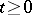, identical in law, also the so-called inverse local time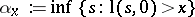and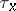are identical in law. Hence, the finite-dimensional distributions ofare determined by the Laplace transform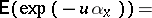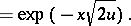The mapping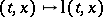,,, is continuous. This is due to H.F. Trotter [a12]; for a proof based on the Itô formula, see, e.g., [a3].

The behaviour of the process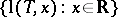can be characterized for some stopping times(for first hitting times, for instance; cf. also Stopping time). Results in this direction are called Ray–Knight theorems [a10], [a5]; see also [a2].

The process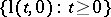is an example of an additive functional of Brownian motion having support at one point (i.e. at). As such it is unique up to a multiplicative constant. See [a1].

Brownian local time is an important concept both in the theory and in applications of stochastic processes. It can be used, e.g., to construct diffusions from Brownian motion via random time change and to analyze stochastic differential equations (cf. also Stochastic differential equation). There are some natural problems in stochastic optimal control (finite fuel problem) and in financial mathematics (barrier options), for instance, where (Brownian) local time plays a crucial role.

For a survey article, see [a8].

How to Cite This Entry:
Brownian local time. Encyclopedia of Mathematics. URL: http://encyclopediaofmath.org/index.php?title=Brownian_local_time&oldid=13305
This article was adapted from an original article by Paavo Salminen (originator), which appeared in Encyclopedia of Mathematics - ISBN 1402006098. See original article